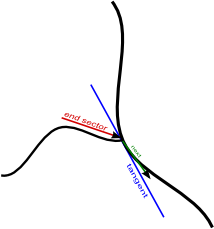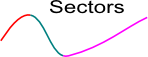## NAME

r.stream.segment - Divides network into near straight-line segments and calculate its order.

## KEYWORDS

raster, hydrology, stream network, stream divide

## SYNOPSIS

r.stream.segment
r.stream.segment --help
r.stream.segment [-rm] stream_rast=name direction=name elevation=name segments=name sectors=name [length=integer] [skip=integer] [threshold=float] [memory=integer] [--overwrite] [--help] [--verbose] [--quiet] [--ui]

### Flags:

-r
Output angles in radians (default: degrees)
-m
Use memory swap (operation is slow)
--overwrite
Allow output files to overwrite existing files
--help
Print usage summary
--verbose
Verbose module output
--quiet
Quiet module output
--ui
Force launching GUI dialog

### Parameters:

stream_rast=name [required]
Name of input raster map with stream network
direction=name [required]
Name of input raster map with flow direction
elevation=name [required]
Name of input elevation raster map
segments=name [required]
Name for output vector map to write segment attributes
sectors=name [required]
Name for output vector map to write sector attributes
length=integer
Search length to calculate direction
Must be > 0
Default: 15
skip=integer
Skip segments shorter than
Must be >= 0
Default: 5
threshold=float
Max angle (degrees) between stream segments
Must be > 0
Default: 160
memory=integer
Max memory used in memory swap mode (MB)
Default: 300

## OPTIONS

-r
Directions and azimut output in radians. Default is degrees.
-m
Only for very large data sets. Use segment library to optimize memory consumption during analysis
stream_rast
Stream network: name of input stream map. Streams shall be ordered according one of the r.stream.order ordering system as well as unordered (with original stream identifiers) Because streams network produced by r.watershed and r.stream.extract may slightly differ in detail, it is required to use both stream and direction map produced by the same module. Stream background shall have NULL value or zero value. Background values of NULL are by default produced by r.watershed and r.stream.extract. If not 0 or NULL use r.mapcalc to set background values to null.
direction
Flow direction: name of input direction map produced by r.watershed or r.stream.extract. If r.stream.extract output map is used, it only has non-NULL values in places where streams occur. NULL (nodata) cells are ignored, zero and negative values are valid direction data if they vary from -8 to 8 (CCW from East in steps of 45 degrees). Direction map shall be of type CELL values. Region resolution and map resolution must be the same. Also stream_rast network map must have the same resolution. It is checked by default. If resolutions differ the module informs about it and stops. Region boundary and maps boundary may be differ but it may lead to unexpected results.
elevation
Elevation: name of input elevation map. Map can be of type CELL, FCELL or DCELL. It is not restricted to resolution of region settings as stream_rast and direction.
length
Integer values indicating the search length (in cells) to determine straight line. The longest length parameter the module treats more tolerant local stream undulation and inequalities. Default value of 15 is suitable for 30 meters DEMs. More detail DEMs may requre longer length.
skip
Integer values indicating the length (in cells) local short segment to skip and join them to the longer neighbour. The shortest length parameter the more short segments will be produced by the module due to undulation and inequalities. Default value of 5 is suitable for 30 meters DEMS. More details DEMS may require longer length.
threshold
real value indicates the internal angle between upstream and downstream direction to treat actual cell as lying on the straight line. Greater values (up to 180 degrees) produce more segments. Lower values produce less segments. Values below 90 in most cases will not produce any additional segments to those resulting from ordering.

## OUTPUTS

The module produces two vector maps: one representing original segments (where a segment is a streamline where its order remains unchanged) and the second divided into near straight line sectors resulting form segmentation process. Most of the segment and sectors attributes are the same as in r.stream.order vector output.

segments
Vector map where every segment has its own category and following attributes:
• segment: integer, segment identifier
• next_segment: integer, topological next segment identifier
• s_order: integer, segment order
• next_order: integer, topological next segment order
• direction: double precision, full segment direction (0-360)
• azimuth: double precision, full segment azimuth (0-180)
• length: double precision, segment length
• straight: double precision, length of straight line between segment nodes
• sinusoid: double precision, sinusoid (length/straight)
• elev_min: double precision, minimum elevation (elevation at segment start)
• elev_max: double precision, maximum elevation (elevation at segment end)
• s_drop: double precision, difference between start and end of the segment
• out_direction: double precision, direction (0-360) of segment end sector
• out_azimuth: double precision, azimuth (0-180) of segment end sector
• out_length: double precision, length of segment end sector
• out_drop: double precision, drop of segment end sector
• tangent_dir: double precision, direction of tangent in segment outlet to the next stream
• tangent_azimuth: double precision, azimuth of tangent in segment outlet to the next stream
• next_direction: double precision, direction of next stream in join with current segment
• next_azimuth: double precision, azimuth of next stream in join with current segmentsectors
Vector map where every sector has its own category and following attributes:
• sector: integer, sector category
• segment: integer, segment category (to establish relationship)
• s_order: integer, segment order
• direction: double precision, sector direction
• azimuth: double precision, sector azimuth
• length: double precision, sector length
• straight: double precision, length of straight line between sector nodes
• sinusoid: double precision, sinusoid (length/straight)
• elev_min: double precision, minimum elevation (elevation at sector start)
• elev_max: double precision, minimum elevation (elevation at sector end)
• s_drop: double precision, difference between start and end of the sectorRelation between segments and sector may be set up by segment key.

The main idea comes from works of Horton (1932) and Howard (1971, 1990). The module is designed to investigate network lineaments and calculate angle relations between tributaries and its major streams. The main problem in calculating directional parameters is that streams usually are not straight lines. Therefore as the first step of the procedure, partitioning of streams into near-straight-line segments is required.

The segmentation process uses a method similar to the one used by Van & Ventura (1997) to detect corners and partition curves into straight lines and gentle arcs. Because it is almost impossible to determine exactly straight sections without creating numerous very short segments, the division process requires some approximation. The approximation is made based on three parameters: (1) the downstream/upstream search length, (2) the short segment skipping threshold, and (3) the maximum angle between downstream/upstream segments to be considered as a straight line. In order to designate straight sections of the streams, the algorithm is searching for those points where curves significantly change their direction. The definition of stream segments depends on the ordering method selected by the user, Strahler's, Horton's or Hack's main stream, or the network may remain unordered. All junctions of streams to streams of higher order are always split points, but for ordered networks, streams of higher order may be divided into sections which ignore junctions with streams of lower order. In unordered networks all junctions are always split points. In extended mode the module also calculates the direction of a stream to its higher order stream. If the higher order stream begins at the junction with the current stream (Strahler's ordering only) or if the network is unordered, the direction is calculated as the direction of the line between junction point and downstream point (Howard 1971) within the user-defined global search distance. If a higher order stream continues at the junction, its direction is calculated as the direction of the tangent line to the stream of higher order at the junction point. To avoid local fluctuation, the tangent line is approximated as a secant line joining downstream/upstream points at a distance globally defined by the search length parameter (1). Such a definition of the angle between streams is not fully compatible with Horton's original criterion.

## NOTES

The module can work only if direction map, stream_rast map and region have the same settings. It is also required that stream_rast map and direction map come from the same source. For lots of reason this limitation probably cannot be omitted. This means that if stream_rast map comes from r.stream.extract, also direction map from r.stream.extract must be used. If stream_rast network was generated with MFD method, also MFD direction map must be used.

## EXAMPLE

```g.region -p -a raster=elevation
r.watershed elevation=elevation threshold=10000 drainage=direction stream=streams
r.stream.order stream_vect=streams direction=direction strahler=riverorder_strahler
r.stream.segment stream_rast=riverorder_strahler direction=direction \
elevation=elevation segments=river_segment sectors=river_sector
```

r.watershed, r.stream.extract, r.stream.order, r.stream.basins, r.stream.slope, r.stream.stats, r.mapcalc,

## REFERENCES

Horton, R. E., (1932). Drainage basin characteristics: Am. Geophys. Union Trans., (3), 350-361.

Howard, A.D. (1971). Optimal angles of stream junction: Geometric, Stability to capture and Minimum Power Criteria, Water Resour. Res. 7(4), 863-873.

Howard, A.D. (1990). Theoretical model of optimal drainage networks Water Resour. Res., 26(9), 2107-2117.

Van, W., Ventura, J.A. (1997). Segmentation of Planar Curves into Straight-Line Segments and Elliptical Arcs, Graphical Models and Image Processing 59(6), 484-494.

r.mapcalc, r.stream.channel, r.stream.distance, r.stream.extract, r.stream.order, r.stream.slope, r.stream.snap, r.stream.stats, r.watershed